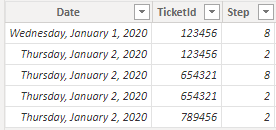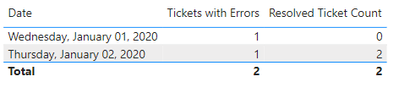cancel
Showing results for
Did you mean:Helper I

## Calculate values with same ID, but different secondary attribute

Hello!

I have a table called Logging. There columns Date, TicketId, Step and Result. Some TicketID have a Result = "Error", Step = 8, and if it was fixed later on, it will recieve new row with Result = "Successfull" and Step = 2.

 Date TicketId Step Result 01.01.2020 123456 8 Error 01.01.2020 123456 2 Successfull 02.01.2020 654321 8 Error 02.01.2020 654321 2 Successfull

There is also matrix with calculated measure that counts unique TicketId with Step = 8.

 Date Errors_dist 01.01.2020 1 02.01.2020 1

I need to calculate new measure to look on TicketID with error, take this ID, look on its own table Logging ones again and try to find this ID with a Status = "Successfull".

 Date Errors_dist Solved_errors 01.01.2020 1 1 02.01.2020 1 1

The measures to calculate TicketID with errors look like this:

First: LogDistcount_ = DISTINCTCOUNT(Logging[TicketId])

Second: Errors_dist = CALCULATE(Logging[LogDistcount_], Logging[Step] = 😎

1 ACCEPTED SOLUTIONSolution Sage

I started with the dataset...I created a calculated column...

`Resolved Tickets = var _isErrorTicket =CALCULATE(MAX('Table (2)'[Step]), ALLEXCEPT('Table (2)', 'Table (2)'[TicketId]))var _calc =IF(    AND('Table (2)'[Step] = 2, _isErrorTicket = 8),    1,    0)Return_calc`

And then wrote the measures...

`Tickets with Errors = CALCULATE(    DISTINCTCOUNT('Table (2)'[TicketId]),    'Table (2)'[Step] = 8)`
`Resolved Ticket Count = SUMX('Table (2)', 'Table (2)'[Resolved Tickets])`

And ended up with...Hope this gets you pointed in the right direction.

3 REPLIES 3Solution Sage

I started with the dataset...I created a calculated column...

`Resolved Tickets = var _isErrorTicket =CALCULATE(MAX('Table (2)'[Step]), ALLEXCEPT('Table (2)', 'Table (2)'[TicketId]))var _calc =IF(    AND('Table (2)'[Step] = 2, _isErrorTicket = 8),    1,    0)Return_calc`

And then wrote the measures...

`Tickets with Errors = CALCULATE(    DISTINCTCOUNT('Table (2)'[TicketId]),    'Table (2)'[Step] = 8)`
`Resolved Ticket Count = SUMX('Table (2)', 'Table (2)'[Resolved Tickets])`

And ended up with...Hope this gets you pointed in the right direction.Helper I

@jgeddes  Everything worked, thank you! But, I forgot to mention. In the table could be duplicates with TicketID and the same Step number. For example, TicketID 123456 with a Step = 2 can meet in the table 4 times and the formula Resolved Ticket Count will sum up all 4. But how to calculate distinct? Only 1 of all these 4?Solution Sage

This should do it...

`Resolved Ticket Count = CALCULATE(    DISTINCTCOUNT('Table (2)'[TicketId]),    'Table (2)'[Resolved Tickets] = 1)`